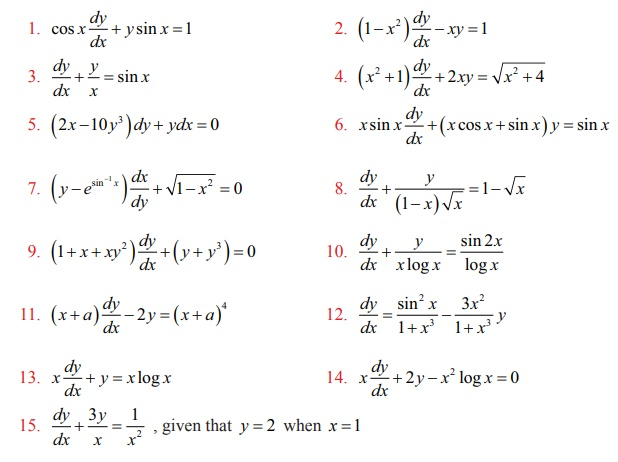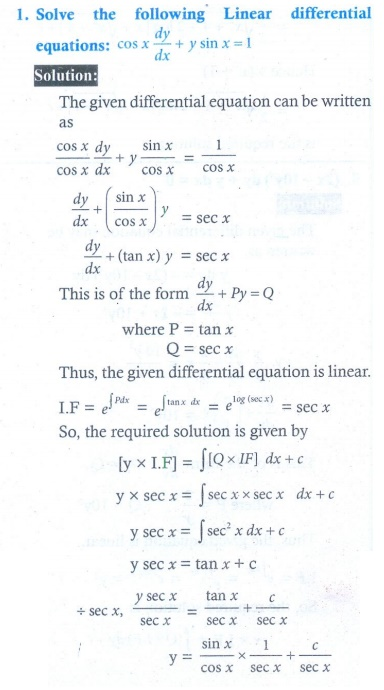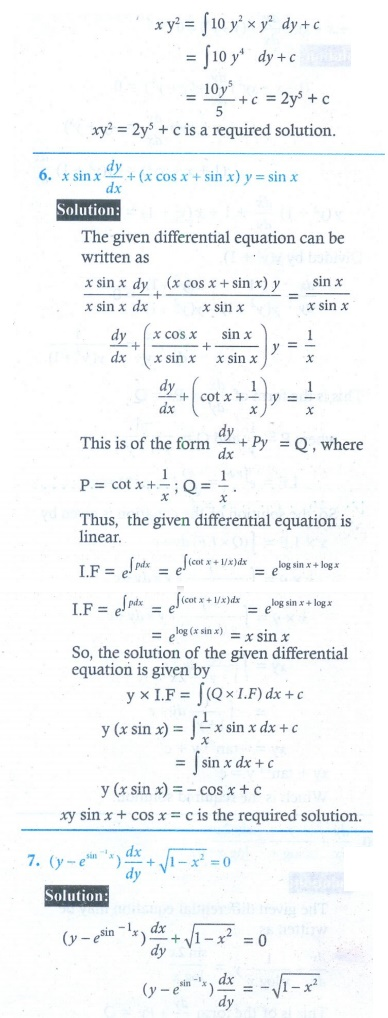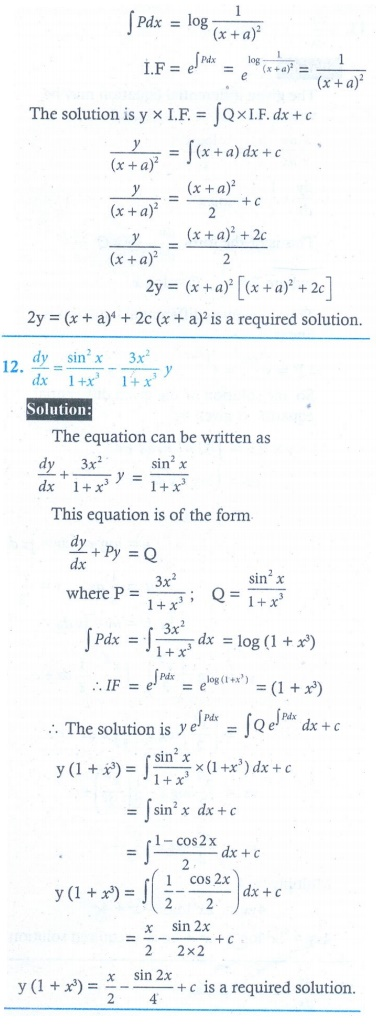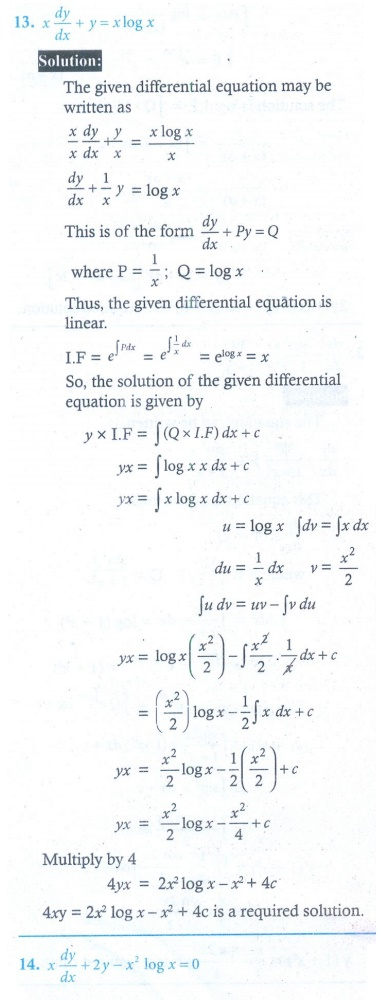Home | | Maths 12th Std | Exercise 10.7: First Order Linear Differential Equations

# Exercise 10.7: First Order Linear Differential Equations

Maths Book back answers and solution for Exercise questions - Mathematics : Ordinary Differential Equations: First Order Linear Differential Equations - Exercise Problem Questions with Answer, Solution

EXERCISE 10.7

Solve the following Linear differential equations: